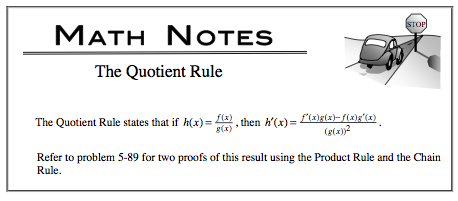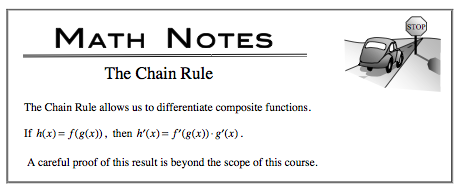### Home > CALC > Chapter 5 > Lesson 5.2.5 > Problem5-106

5-106.

Use your derivative tools to find the second derivative, $\frac { d ^ { 2 } y } { d x ^ { 2 } }$, of each function below.

1. $y = \frac { \operatorname { sin } x } { x }$1. $y =\operatorname{csc}^2 x −\operatorname{cot}^2 x$1. $y = \sqrt { \frac { 1 } { x } }$

You could use the quotient rule AND the chain rule...Or you could rewrite $y$ as with exponents and quickly use the Power Rule!

1. $y = | x - 2 |$

Rewrite $y$ as a piecewise function. The derivative will be a piecewise function as well. So will the second derivative.